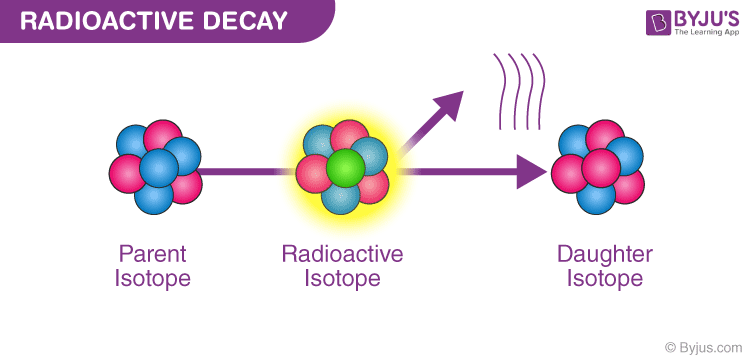Radioactivity is the phenomenon exhibited by the nuclei of an atom as a result of nuclear instability. In the year 1896, Henry Becquerel discovered this phenomenon. Radioactivity is a process by which the nucleus of an unstable atom loses energy by emitting radiation. In a drawer which was having photographic plates, a small amount of Uranium compound was wrapped in a black paper and was kept in it. Examination of these plates later resulted that there has been an exposure. This phenomenon came to be known as Radioactive Decay. Radioactive elements are the elements or isotopes which emit radiation and undergo the process of radioactivity. In this article, let us learn about radioactive decay law in detail.

The atom particles cannot be bounded because there is no energy due to the presence of an unstable nucleus in the element’s radioisotope. There is a constant decay in the isotopes in order to stabilise itself with a release of a lot of energy in the form of radiations.

Transmutation is referred to as the process of isotope transforming into an element of a stable nucleus. It can occur both in natural or artificial ways.There are three types, namely:

1. Alpha
2. Beta
3. Gamma.

### 1. Alpha decay:

When an alpha particle emits its nucleus, the process is called alpha decay. The formula of alpha decay is given as:

 $$E=(m_{i}-m_{f}-m_{p})c^{2}$$

Where,

• mi is the initial mass of the nucleus
• mf is the mass of the nucleus after particles emission
• mp is the mass of the emitted particle

The nucleus of helium is taken as the alpha particle which is very stable. It has a group of two protons and two neutrons. For example, alpha decay of uranium-238 is shown below

-$$U_{92}^{238}\rightarrow Th_{90}^{234}+He_{2}^{4}$$

Transmutation is referred to as the process of isotopes transforming into an element of a stable nucleus.

### 2. Beta Decay:

A beta particle is often referred to as an electron, but it can also be a positron. If the reaction involves electrons, nucleus shed out neutrons one by one. Even the proton number increases accordingly. A beta decay process is shown below:

$$Th_{90}^{234}\rightarrow Pa_{91}^{234}+e_{-1}^{0}$$

### 3. Gamma Decay:

The nucleus has orbiting electrons which indeed have some energy, and when an electron jumps from a level of high energy to a level of low energy, there is an emission of a photon. The same thing happens in the nucleus: whenever it rearranges into a lower energy level, a high-energy photon is shooted out which is known as a gamma ray.

In the sample, there is a proportionality between radioactive decays per unit time and the overall number of nuclei of radioactive compounds. We can mathematically quantify the rate of this type of decay through this proportionality.

If N = number of nuclei in a sample and
$$\Delta N$$ = number of radioactive decays per unit time $$\Delta t$$ then,
$$\Delta N\Delta t\propto N$$ or
$$\Delta N\Delta t = \lambda N$$,
Where,
$$\lambda$$ = constant of proportionality ( or radioactive decay constant or disintegration constant).

Also, $$\Delta N$$ is the reduction of the total number of nuclei present in the sample.

According to convention, this should be termed negative.
$$\partial N\partial t=-\lambda N$$

After rearranging this,
$$\partial NN=-\lambda \partial t$$

Integrating both sides will results in,
$$\int NN_{0}\partial NN=-\lambda \int tt_{0}\partial t$$ $$InN-InN_{0}=-\lambda \left ( t-t_{0} \right )$$

Here,
$$N_{0}$$ represents the original number of nuclei in the sample at a time

$$t_{0}$$, i.e. t=0.

Applying that in the equation results in;
$$InNN_{0}=-\lambda t$$

$$N_{t}=N_{0}^{e-\lambda t}$$

This type of decay is exponential.

Rate of Decay: From the above expression, the focusing on the rates not on the number. Rate here is the change per time.

Calculating the rate of decay,
$$R=-\partial N\partial t$$

Substituting $$N_{t}$$ in the equation and differentiating it,
$$N_{t}=N_{0}^{e-\lambda t}$$

Differentiation result is,
$$R=-\partial N\partial t=\lambda N_{0}^{e-\lambda t}R=R_{0}^{e-\lambda t}$$ $$R_{0}$$

here represents the decay rate at time, t=0.

Substituting the original equation back here,
$$\Delta N\Delta t=\lambda N$$

We get,
$$R=\lambda N$$

The total decay rate R of a radioactive sample is called the activity of that sample which is represented with the unit Becquerel, in the honour of its scientist. 1 becquerel = 1 Bq = 1 decay per second Another unit is the curie. 1 curie = 1 Ci = 3.7×$$10^{10}$$Bq

### Law Of Radioactive Decay Derivation

According to the radioactive decay law, when a radioactive material undergoes either 𝛼 or β or ℽ decay, the number of nuclei undergoing the decay per unit time is proportional to the total number of nuclei in the given sample material.

The radioactive decay law states that “The probability per unit time that a nucleus will decay is a constant, independent of time”.

It is represented by λ (lambda) and is called decay constant.

The mathematical representation of the law of radioactive decay is:

$$\frac{\Delta N}{\Delta t}\propto N$$

Where,
N: the total number of nuclei in the sample Δ
N: number of nuclei that undergoes decay
$$\Delta t$$: unit time

$$\frac{\Delta N}{\Delta t}=\lambda N$$ (eq.1)

Where,
λ: radioactive decay constant also known as disintegration constant

The change in the sample with respect to the number of nuclei is given as:
$$\frac{dN}{dt}=-\lambda N$$ $$\frac{dN}{N}=-\lambda dt$$ $$\int_{N_{0}}^{N}\frac{dN}{N}=\lambda\int_{t_{0}}^{t}dt$$ (eq.2)
$$lnN-lnN_{0}=-\lambda (t-t_{0})$$ (eq.3)

Where,
$$ln(\frac{N}{N_{0}})=-\lambda t$$ $$N(t)=N_{0}e^{-\lambda t}$$ (eq.4)